## Wednesday, 30 December 2009

### Counting Spanning Trees, A Neat Approach

In my last blog I commented on the relationship between a "parking sequence" problem for n cars and the number of spanning trees of degree n+1. Because I knew so little about the "WHY" of the counting method, I decided to read up a little on the proof for counting spanning trees. It turns out that Cayley [A. Cayley. A theorem on trees. Quart. J. Math., 23:376–378, 1889.] first proved the sequence, but the first combinatorial type proof was by Prufer in 1918 [H. Prufer. Never beweis eines satzes ¨uber permutationen. Arch. Math. Phys. Sci., 27:742–744,1918.] Prufer's method was so interesting, I thought I would share it with interested others who might not have come across it.

Prufer proved that the number of spanning trees for n nodes would be nn-2 by showing that there is a bijection (For my students, you can think of that as sort of like a one-to-one function relationship) between the set of spanning trees and the number of ordered vectors (don't think that is how he said it) of length n-2 of the integers from one to n. For example, if n = 5, then the number of ordered triples (5-2) you could make with the integers 1 through 5 (allowing repetition) would count the number of spanning trees. To do it, he had to show that each spanning tree would produce a unique ordered n-2 tuple (is that a math word?) and that each such vector would produce a unique spanning tree.... and then he did... and since there are quite easily n choices for each of the n-2 spaces, the number of spanning trees must equal nn-2.... admit it, that's pretty neat..

For a Spanning tree such as the one belowthe rules for deriving a code is to take the leaf (vertex with only a single edge to it) with the smallest number, and remove it, and record the vertex it is connected to. In the image, we would remove vertex 1, and write a 5. Now the lowest number on a leaf is 3, and it is connected to vertex 2, so we remove the vertex at 3 and our code is now 5,2. The next lowest leaf is 4, and it is also connected to vertex 2, so our code is now 5,2,2. At this point we have the n-2 terms we need for degree five.. and we can stop... I did some serious head scratching at this point about how he knew that we could ignore the remaining two vertices... but somehow, it all works... you can take the code and presto, you get all the vertices and edges...

Let's make up a code and see what happens... if we write (2,3,3) what spanning tree would it produce. Well, we know the degree of each vertex is one more than the number of times it appears on the code, so 1, 4, and 5 have degree 1 (they are leaves) and 2 has degree 2. Vertex 3 has degree 3, since it shows up 2 times in the code. We begin by remembering that the lowest numbered leaf (one) was removed first, so one must be connected to the vertex named first in our code, 2. Our next smallest leaf is vertex 4, so it must be connected to the vertex named second in the code, vertex 3. Finally we have vertex 5 as a leaf, and so we must have it connected to vertex 3 as well. Finally, we need to remember that the numbers in the code were connected to each other (hence the additional degree) and we should connect vertices 2 and 3.But what if we changed the code to (3,2,3)? Same numbers, different order... We still have the same three leaves at 1, 4, and 5, but now 1 is connected to vertex 3; 4 connected to 2; and 5 would be connected to three.What I haven't figured out yet (any help out there??) is how to find a bijection between the Prufer codes and the parking problem. More to learn....

## Sunday, 27 December 2009Here are two interesting problems, the second is a little more abstract, but bare with me... there is a purpose...

Suppose there are n parking spaces in a row along a one way street, and n people are driving into town, each with a most preferred spot in the row of spaces. Each of the n people adopts the plan that they will drive along the line until they come to their preferred space. If it is open, they take it, and if not, they continue driving and will take the next available spot. For instance if n=2 parking spaces, then the two drivers would find a parking space if both wanted the first spot, or they both wanted different spots; but one of them is out of luck if they both preferred the last spot, since the one who got there second is out of parking places. Thus for n=2, there are 3 possible solutions out of 2^2 = 4 possible situations. So here are the easy questions, find the number of possible solutions for n=3 and 4, and their associated probabilities of success.
And for the clever people, generalize for n.

If you found that either too hard, too easy, or just want to read on, here is a second problem. For those who have never studied graph theory, or don't remember the terms, a brief introduction. A complete graph with n nodes is called the k_n graph. For n = 4 and 5 the complete graphs look like the ones shown below.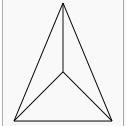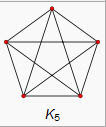Now a spanning Tree is a graph with N vertices but no complete circuits. You can get from any vertex to any other, but only by one path. Here is an example of a tree with six labeled vertices.Finally, we can give the question... For a complete graph of 3 vertices, 4 vertices, or n vertices, how many different spanning trees are possible?

The amazing result is that the two problems seem to be exactly the same, at least they produce the same sequence. The number of solutions for the parking problem is 1, 3, 16, 125, ... (N+1)(n-1)... For the spanning trees, if we start with a graph of 2 points, there is only one tree. For three points there can be three different trees, and for four... yeah..16 . The image below shows all 16 of them as depicted by Wolfram Mathworld.. the sequence is the same but the index is off by one, so we get 1, 3, 16, 125, ... (n)(n-2)... Ok, here is the real question for this blog... why are they the same...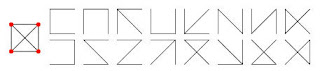Ok, one more curiously beautiful detail about this problem.... the probability of success for the n parking cars at the top has a limit of e/(n+1) as n approaches infinity. I love how often e pops up in these "sorting" type problems.

## Friday, 25 December 2009

### Merry Christmas

Stole this off John's Post at The Endeavour, Well worth repeating

## Wednesday, 23 December 2009

### Exploring an Isoperimetric Theme

Recently we have been doing maxima/minima applications in calculus. By the time they get her most of them know that when they maximize the area of a rectangle with perimeter constraints, they get a square. In Pre-calc we do some basic problems with 2 adjacent pens, a single pen against a wall or river, and all the similar cases. I have written about the generalizations of some of these here, and here. The problem of two adjacent congruent pens is (or should be) easy for a calculus or precalc student.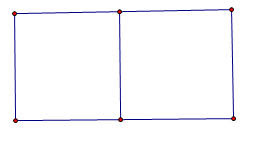It turns out that the maximum occurs when the total width is one-fourth the perimeter and the height is one-sixth the perimeter, for a maximum area of one twenty-fourth of the perimeter value (in square units, of course). If you extend that to even more congruent rectangular pens placed end to end it turns out that the width stays the same?

If you extend this, as many teachers do, to a shape like this one, it starts to get interesting to the more observant student.The height is now one-eighth of the total , and the width is one-sixth of the perimeter. Even slow students can figure out that the area of the total turns out to be one forty-eighth of the value of the perimeter. Some will even generalize the area of an array of mxn congruent pens to be the perimeter squared divided by [4(m+1)(n+1))].... but it seems to take forever for some one to finally realize that in all these problems, whether there was one rectangle, or one with one side adjacent to a river or barn, or rows of rectangles... there is one invariant...

Theorem one for rectangular arrays of congruent rectangular pens "The amount of fence going right to left is the same as the amount going up and down." If you want to maximize a rectangular array with five rows of three pens in each row, we will need 24 lines of fence, four going vertically, six going horizontally. Use half the fencing to make the four vertical fences, and the other half to make the other six going horizontally. (Prove..... dear reader) ..

I always thought this fifty-fifty use of fencing was a cute mathematical "teacher trick" but I have recently come to believe there is something deeper at work. I played around recently with this problem but loosened the constraints on the pens to be just equal area rectangles rather than congruent. This offered some nice areas to explore. Which would be better, three congruent pens in a row, or two pens with a third beneath... like so.The three adjacent pens turn out to have a max area when the width was p/4 (where p is the perimeter) and the height was p/8, giving a maximum area of p2/32... and as before, that mean that half the perimeter was used for the vertical sections, and half for the horizontal sections..and for the three non-congruent sections with equal area the width was p/6, and the length was 3P/16, giving a max area of....wait, that's also p2/32. (Ok, I hadn't expected that)... but when I checked the dimensions, it turns out that the horizontal sections use up exactly half the perimeter, and the vertical sections the other half in both cases.... hmmm...

Ok, but every kid knows that the area is maximized when the rectangle is closest to a square... so what if we just put three squares arranged as shown below and didn't require that the whole assembly forms a rectangle... I thought that would probably be even more area..It turns out that it isn't, The equal distribution of left and right still works out, but the total area is only 3p2/100 or about 96% of the other shapes. (ok, I wasn't ready for that)...

So, I decided to move up to five pens... I passed over four absolutely sure that the division into four equal squares in a two by two array would be the most efficient, but five offered several variations, five in a row; two rows of two with a single in the third row, or three in one row and two in another... The five congruent squares in a row gave a max area of p2/48, but the set up with three pens in one row and two in another gave a slightly larger area of 5p2/216 or p2/43.2. The 2,2,1 set up (shown below) gave not quite the same area..This set up gave a max area of p2/44.8.

From all of this I have a formed a totally unproven conjecture about the maximum area when n equal area rectangular pens are set out in a rectangular boundary with a given amount of fencing.
The Theorem One above for congruent rectangles seems to be a stronger property than I suspected, and is applicable to all equal area pens in a rectangular boundary, And the conjecture is that when the number of pens, n, is between x2-x and x2+x, the maximum area will be found when there are x-rows of pens and all of the pens have either x-1, x, or x+1 pens in the row.

The number of pens in any row will never differ by more than one from any other row. For values of n that are perfect squares or pronic (product of consecutive integers), the number of pens in each row will be the same. When n > x2 there will n-x2 rows that have x+1 pens, and the remaining rows will all have x pens. Similarly, if n is less than x2 there will be x2 - n rows that have x-1 pens and the remaining rows will have x pens each. As an example, if n= 23, then x=5 since 52-5 is less than 23<52+5. And for 23 pens there will be five rows with x=five pens in three of them, and x-1=four pens in two of them.

I also have discovered that the theorem about equal lengths of fencing horizontally and vertically seems to be more important as a "rule of thumb" for maximizing than is equal sides. For example if five squares are set out as efficiently as possible (four in a square with one attached to a side), the area will be p2 / 45 with 8/15 of the perimeter going one way(say horizontally) and 7/15 going the other. If we change the squares to rectangles with each having a height of p/16 and a width of p/14 the area is p2/44.8.. an improvement of about 1/2 %....
Even if you go to six pens (where there is no lost perimeter enclosing the odd pen), putting two rows of three squares gave me an area of P2 / (48 1/3) but using the equal division of fencing gives the very slightly improved maximum of p2/48.

I can't explain why, but the equal division of fencing theorem is stronger than I first suspected, and if someone out there can shed more light on it than my feeble exploration, please do.

I haven't had time to expand the formula for the max area of all n when a rectangle is divided into congruent area rectangles, (not too difficult, I think).. but for square or pronic numbers of pens, it seems the max area will always be p2/(4 (x+1)(y+1)) where xy=n. As a limit as n approaches infinity, it seems the max area for rectangles divided into n equal area rectangles approaches### Learning Styles Theory BOGUS?

Hat tip to Steve Phelps who put me on to this article... Matching Teaching Style to Learning Style May Not Help Students.

Some clips from the article:

"There is no strong scientific evidence to support the "matching" idea, they contend in a paper published this week in Psychological Science in the Public Interest. And there is absolutely no reason for professors to adopt it in the classroom."

"Lots of people are selling tests and programs for customizing education that completely lack the kind of experimental evidence that you would expect for a drug," Mr. Pashler says. "Now maybe the FDA model isn't always appropriate for education—but that's a conversation we need to have."

I think the report trys to be balanced... 'Advocates of learning styles respond that Mr. Pashler is the one who lacks evidence. Robert J. Sternberg, dean of arts and sciences at Tufts University and a psychologist who has done a lot of work on learning styles, says in an e-mail message to The Chronicle that the researchers did not fully survey the scholarly literature, and thus "come across looking either biased about or largely ignorant of the field."'

Read and draw your own conclusions...but the one line that resonated the most with me is this one...."For a given lesson, one instructional technique turns out to be optimal for all groups of students, even though students with certain learning styles may not love that technique." Lesson (for this teacher) Let the lesson you are teaching drive the instructional strategy.

## Sunday, 20 December 2009

### Puzzle Master Scott Kim

For all the kids who fiddle around with the puzzles in my classroom, and those who wish they could...

## Saturday, 19 December 2009

Mathematics of origami..... flapping birds and space telescopes, and geometry like you OUGHT to see it..... For all my students who think geometry is disposable

## Friday, 18 December 2009

### Exponential growthMy Senior math kids are studying exponential curves now, and their inverses, logarithmic curves... it seems hard to get across how fast they grow, but then you flick on The Endeavor, and he has this cartoon from Abstruse Goose... Sometimes a cartoon is the best teaching tool...
Thanks John...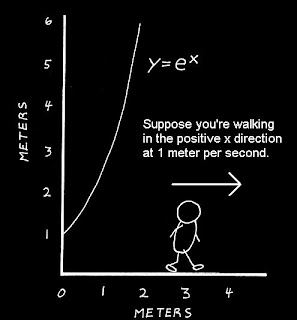## Wednesday, 16 December 2009

Recently someone on the Calculus EDG asked about the value of. I sent a link to some work I had done a while ago exploring the same idea, and extending to finding the value of. I have picked out some parts below, but you can see the rest at this link. This is a very old Word Document so give it some time to load. Hopefully it is worth while. Dave Renfro then sent me a copy of some papers about the topic, including this one from a 1935 American Mathematical Monthly.

When you take the iterated square root of a number, such as $x = \sqrt{n+ \sqrt{n+ ...}}$ and then square both sides, you get $x^2 = x + n$. This means that we can find solutions using basic quadratic solution approaches, and then find solutions that produce integer values of x. The positive solution becomes $\frac{\sqrt{4n+1}+1}{2}$

One of the nice things I discovered was that the iterated square roots of 2 was not the only number that gave an integer answer. In fact, 2, 6, 12, 20, 30.... all were equal to integer values... This sequence is the pronic or oblong numbers, which are twice the triangular numbers. These numbers can be expressed as (n)(n+1) or n,sup>2 + n. It took me a moment to realize why they are the ones that would work. These are numbers that, when multiplied by four and increased by 1, become perfect squares, $4 (n^2+n)+ 1 = 4n^2 + 4n+1 = (2n+1)^2$. And the square root, being an odd (2n+1) number so that when 1 is added, we get a number divisible by 2.

It seems, according to the Herschfeld article, that the problem was a common topic in the Columbia classes of Dr. Edward Kasner. Kasner, of course, is known for his part in the creation of the term "googol" for 10^100. If your interested in any of these topics, check either or both the links above .

I had not yet tried to consider the roots of the cube root of (a+cube root(a+ .... etc)) and so I wanted to take a shot.. By the same process I had used before, the value would be the solution to x^3-x-n=0 .

If the iterated value was 1, the value approaches about x=~1.32472. For n=2 the value is x=~1.52138. By the time we get to n=6, we get x=2. The actual solution for any n isOK... that really isn't very much fun to play with, but after some experimenting, I came up with the fact that the following sequence of numbers produced integer values when iterated; 6, 24, 120, 210... ; or perhaps it is more revealing to write them a different way (1*2*3) , (2*3*4), (3*4*5)... so they were sort of the three dimensional pronic numbers, the products of three consecutive integers.
I could not manipulate the above equation to make it clear that these were the only values as I had with the quadratic, but it got me thinking, what if I did fourth roots ?
Extending the solutions for square and cube roots, I tried 1*2*3*4 = 24.... but the solution of $n^4 - n- 24=0$ was NOT 2; in fact, it was about 2.1617???
Exploring I found that n=2 was a solution to $n^4 - n- 14=0$. And n=3 was a solution when the constant was 78. The sequence is 14, 78, 252, 620, 1290,... These values follow the form n*(n-1)*(n^2+n+1)
I realized, somewhat belatedly, that you could generate these sequences by simply using nk - n for integer values of k, and factoring the same would give you the simplified form of the expression.
After struggling with solving n^3 - n -k=0 I realized that I could just start with values of n, and find out what k came out to be. A very late "aha" moment. So for n=2, 23 - 2 = ??? and six pops out like Alg I.

There is a familiar quotation about forests and trees that seems to apply here, but it came to me somewhat late.

But sometimes, that's how my mind works... do it the hard way first.

## Tuesday, 15 December 2009

### Folding a cube???

Found this at Math Recreations by Dan MacKinnon. Fold a cube into..well,some interesting stuff.
The origninal work comes from Eric Demaineclick here, then wait, it takes a minute to animate...but well worth it.

## Monday, 14 December 2009

John D. Cook over at the Endeavor always has great stuff, and today his blog led me on a trail to the video below.... only one word...weird... There is no trick, it's just between you and your lying eyes...

## Sunday, 13 December 2009

### Fox Math>

Found this on MediaMatters, where they say it appeared on the December 4 edition of Fox & Friends. You do the math.Kind of goes with their recent Pie-Chart "new-math" approach.

## Saturday, 12 December 2009

### Problem with Unexpected ResultHere is a quick problem with a result which surprised me...

Graph a semi-circle with the center at the origin ... then graph y=2x... The point of intersection is the right angle of a right triangle whose hypotenuse is the diameter. Find the ratio of the legs...

## Wednesday, 9 December 2009

### Fun With Parabolas in Calculus

Ok, two quick mental problems. Some folks will see one as somewhat easier than the other, but ...well heck, read on...

Problem One. A secant cuts the parabola y= x2 at x= -1 and x=1. Find the area of the region between the secant and the parabola..Problem Two. A secant cuts the parabola y= x2 at x= 1+sqrt(5) and x=3+sqrt(5) . Find the area of the region between the secant and the parabola..

Ok, if two seems to hard, try this middle of the road alternate:

A secant cuts the parabola y= x2 at x= 0 and x=2. Find the area of the region between the secant and the parabola..I still find it fascinating each year as I introduce my calculus class to this idea that the answers are all the same. I lead them eventually to conclude that the area between the secant and x2 depends only on the difference between the two x-values.

I was recently reminded of this little parabola property when the very kind Dave Renfro put me on to an article in the December Monthly from the MAA by Bettina and Tom Richmond entitled "How to Recognize a Parabola." Among a dozen really nice properties of parabolas, the first one was this little area relation. They included several that I had used recently in my blog about tangents to a parabola and circle. They also had another theorem closly related to these problems in their eleventh example which says (in my less rigorous language) that the area of the region between the secant and a parabola is 2/3 the area of the parallelogram that inscribes that region. The two ideas together mean that the perpendicualr distance between the secant and the tangent parallel to it when multiplied by the length of the secant is a constant depending only on the difference in the x values also. ... In the region cut off by the horizontal secant the distance from the secant to the vertex is 1 and the length of the secant is 2. Two-thirds their product gives the area of 4/3 sq units. In the region cut off by the secant from x=0 to x=2 the secant has a length of sqrt(20). The tangent parallel to the secant will be at x=1 (that would be Theorem 3 in the Richmonds' paper, "the average of the slopes at the endpoints is the slope at the midpoint" ). The perpendicular distance from the tangent point to the secant line is 1/sqrt(5) , and the product of these two distances is again 2.

The relation between the two above has started me wondering about their tenth theorem, which I had not observed before, and a possible generalization of the theorem. The theorem said that if you took the section formed by a horizontal secant and the parabola, and revolved it around the y-axis, the volume inside the bowl and the volume remaining from a cylinder when the bowl is cut out are the same. Ok... but driving to work I got to wondering, If we took and oblique secant, and rotated the area between the secant and the parabola about the line perpendicular to the secant passing through the point where the tangent would be parallel, would that also have the same volume? (I keep thinking it would, will leave the exercise prove or disprove to the reader).

By the time I get around to writing this, I'm thinking it is NOT the same as the horizontal case... but I keep thinking when I get to it there will be something pretty hiding here...

## Thursday, 3 December 2009

### The Shoestring ExplainedOne of my students got too little sleep last night because he kept trying to figure out why the shoelace algorithm worked. One of those really bright kids who transferred in and just hasn't had an education up to his potential. He has never been introduced to vectors, so after a very cursory overview of the idea of vectors and cross products, I showed him the following as a way of "explanation". He had seen determinants in Alg II but never used to find area.If we take the the set of vertices and add one more point at the origin, then we can draw vectors from the origin to each pair of consecutive vertices. In the first image we have drawn the vetors [1,1] and then [5,2], and we show the triangle area formed between the two vectors. The Determinant of the two vectors will give us twice this area. If we imagine the area being swept out by the first vector rotating until it reaches the second, a rotation of a positive direction will give us a positive value for the determinant, and a negative rotation will give us a negative value. Since we started at (1,1) our first area is negative, and equal toor -3/2.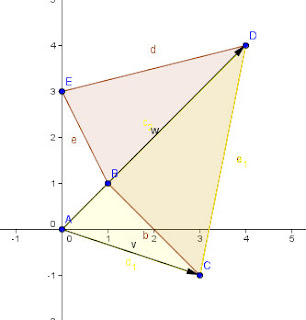As we proceed in order from vertex to vertex, the vector triangles will add and subtract areas as it sweeps around the polygon until it gets back to the starting point. The Vectors from [5,2] ot [4,4] add back the area just subtracted in the first pair of vectors, and also the area inside the polygon formed by triangle BCD (this is just by chance because vectors AB and AD have a common slope)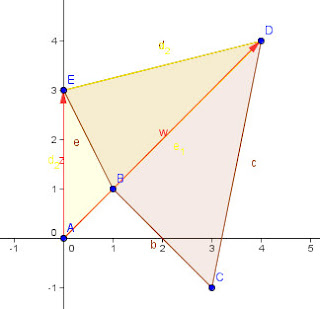The next pair of vectors add the rest of the polygon, but also some excess in the amount of triangle ABE. This excess is removed when we sweep back to the original point A and so we have the final area of the polygon.If we had traversed our way around the vertices in the reverse order, the determinants would have all had their signs reversed and we would have a total which was the negative of the actual area. Hope that is pretty clear, there was a lot more armwaving when I was doing this at the board.

## Wednesday, 2 December 2009

### The Shoelace formula and Formulas for ShoelacesThe shoelace formula is an algorithm for finding the area of a polygon in the plane when the coordinates (x,y) are known.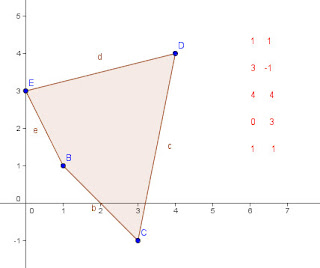For example if the coordinates of a quadrilateral are given as (1,1); (3, -1); (4,4); and (0,3) then the area can be calculated by putting these in two columns (or rows as shown below) and multiplying along diagonals as shown (notice that the first coordinate pair are repeated at the end for ease of computation).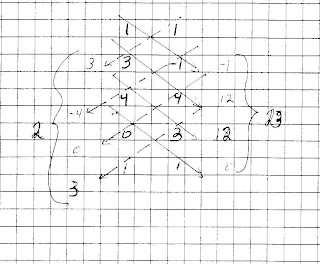The diagonal products to each side are summed, then the absolute value of the difference of the two sums is multiplied by 1/2 to give the area. In this case the right products total to 23, the left to 2, for a difference of 21, so the area is one-half of that, or 10.5 square units. The points have to be taken in order around the polygon in either clockwise or counter-clockwise order using each number once as the first pair. It is the criss-cross appearance of the diagonals that draws the "shoestring" name to the apporach.

The shoelace formula is just a simple diagrammatic extension of an earlier determinant method sometimes (perhaps seldom?) called the Surveyor's formula (not to be confused with the Ptolemaic Egyptian method of the same name for finding areas of quadrilaterals), which does the same computation with a sequence of determinants, like this: Note that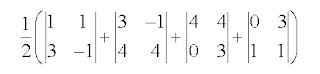This gives ½(-4+16+12-3) which is again the 10.5 sq units we had before. The calculation of each determinant constitute one product from each side of the shoelace algorithm and calculates their difference. (the sum of the differences is the same as the difference of the sums).

Additional illustrations and worked examples can be found at this file by Charles L. Hamberg and Ronald Vavrinek, from the Illinois Mathematics and Science Academy. It also includes a program you can use on your ti-84 or other programmable calculator.

While we are talking about shoelaces, they also showed up in an interesting book by Australian Mathematician Burkard Polster. In "The Shoelace Book", Polster examines various approaches to methods of lacing a pair of shoes, and "mathematizes" lacings formally enough to enumerate the possible lacing paths.. For a shoe with six eyelets on each side there turns out to be 43,200 different paths for a shoelace to pass through every eyelet, even with the added condition that each eyelet must contribute to the essential purpose of pulling the two halves of the shoe together..... go ahead, take a minute and check...I'll wait... dum de dumm..de dumm (all done?) OK.. If that was more difficult than you might have expected, you can find a good link that gives a glimpse of the work at Google Books where he describes lacings by such names as Crisscross, zigzag, bowtie, devil, angel, and star... I'm thinking of switching to the bowtie lacing myself, not just because it is efficient, but I like the look.
You can also order the book from Amazon

Speaking of strings (ok, that's a stretch from the "shoestring" idea) this is blog number 300 on Blogger.... I think I'm a veteran...